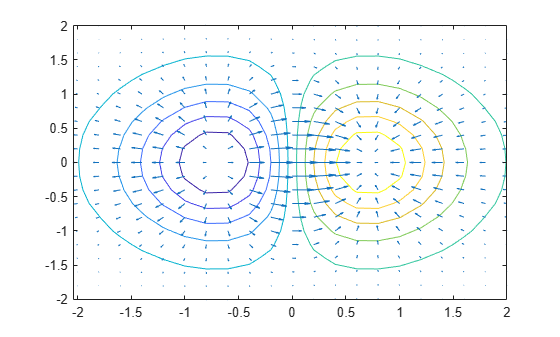# Combine Contour Plot and Quiver Plot

Display contour lines and gradient vectors on the same plot.

Plot 10 contours of $x{e}^{-{x}^{2}-{y}^{2}}$ over a grid from -2 to 2 in the x and y directions.

```[X,Y] = meshgrid(-2:0.2:2); Z = X .* exp(-X.^2 - Y.^2); contour(X,Y,Z,10)```Calculate the 2-D gradient of `Z` using the `gradient` function. The `gradient` function returns `U` as the gradient in the x-direction and `V` as the gradient in the y-direction. Display arrows indicating the gradient values using the `quiver` function.

```[U,V] = gradient(Z,0.2,0.2); hold on quiver(X,Y,U,V) hold off```